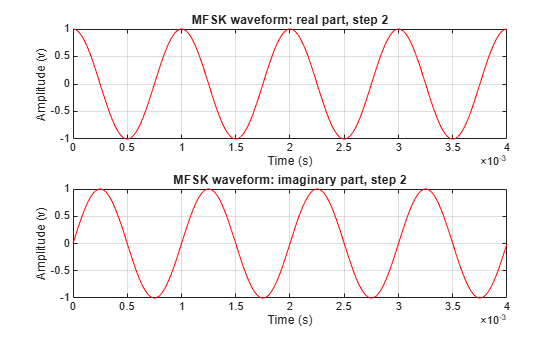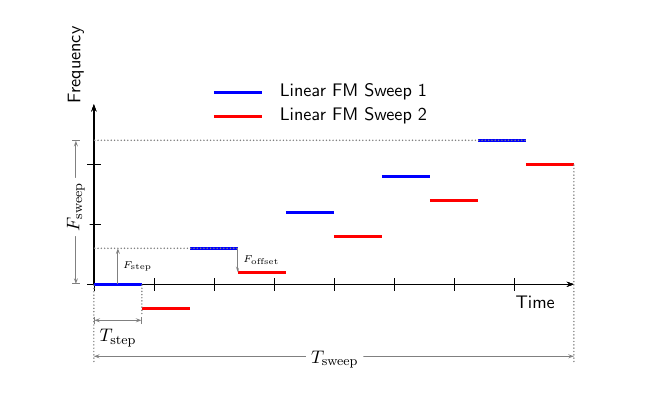# phased.MFSKWaveform

MFSK waveform

## Description

The multiple frequency shift keying (MFSK) waveform is used in automotive radar to improve simultaneous range and Doppler estimation of multiple targets. The `MFSKWaveform` System object™ creates the baseband representation of an MFSK waveform. An MFSK waveform consists of two interleaved sequences of increasing frequencies, as described in Algorithms.

To obtain waveform samples:

1. Define and set up the MFSK waveform. See Construction.

2. Call `step` to generate the MFSK waveform samples according to the properties of `phased.MFSKWaveform`. The behavior of `step` is specific to each object in the toolbox. The output of the `step` method is controlled by the `OutputFormat` property, which has no effect on the properties of the waveform.

Note

Starting in R2016b, instead of using the `step` method to perform the operation defined by the System object, you can call the object with arguments, as if it were a function. For example, ```y = step(obj,x)``` and `y = obj(x)` perform equivalent operations. When the only argument to the `step` method is the System object itself, replace `y = step(obj)` by ```y = obj()```.

## Construction

`sMFSK = phased.MFSKWaveform` creates an MFSK waveform System object, `sMFSK`.

`sMFSK = phased.MFSKWaveform(Name,Value)` creates an MFSK waveform object, `sMFSK`, with additional properties specified by one or more `Name-Value pair` arguments. `Name` must appear inside single quotes (`''`). You can specify several name-value pair arguments in any order as `Name1,Value1,…,NameN,ValueN`.

## Properties

expand all

Sample rate of the signal, specified as a positive scalar. Units are hertz.

Example: 96e6

Data Types: `double`

MFSK sweep bandwidth, specified as a positive scalar. Units are in hertz. The sweep bandwidth is the difference between the highest and lowest frequencies of either sequence.

Example: 9e7

Data Types: `double`

Time duration of each frequency step, specified as a positive scalar in seconds.

Example: 0.2e-3

Data Types: `double`

Total number of frequency steps in a sweep, specified as an even positive integer.

Example: 16

Data Types: `double`

Chirp offset frequency, specified as a real scalar. Units are in hertz. The offset determines the frequency translation between the two sequences.

Example: 500

Data Types: `double`

Output signal grouping, specified as one of `'Steps'`, `'Sweeps'`, or `'Samples'`. This property has no effect on the waveform but determines the output form of the `step` method.

• `'Steps'` — The output consists of all samples contained in an integer number of frequency steps, `NumSteps`.

• `'Samples'` — The output consists of an integer number of samples, `NumSamples`.

• `'Sweeps'` — The output consists of all samples contained in an integer number of sweeps, `NumSweeps`.

Example: `'Samples'`

Data Types: `char`

Number of samples in output, specified as a positive integer. This property applies only when you set `OutputFormat` to `'Samples'`.

Example: 200

Data Types: `double`

Number of frequency steps in output, specified as a positive integer. This property applies only when you set `OutputFormat` to `'Steps'`.

Example: 10

Data Types: `double`

Number of sweeps in output, specified as a positive integer. This property applies only when you set `OutputFormat` to `'Sweeps'`.

Example: 5

Data Types: `double`

## Methods

 plot Plot continuous MFSK waveform reset Reset states of the MFSK waveform object step Samples of continuous MFSK waveform
Common to All System Objects
`release`

Allow System object property value changes

## Examples

collapse all

Construct an MFSK waveform with a sample rate of 1 MHz and a sweep bandwidth of 0.1 MHz. Assume 52 steps with a step time of 4 milliseconds. Set the frequency offset to 1 kHz. There are 4000 samples per step.

```fs = 1e6; fsweep = 1e5; tstep = 4e-3; numsteps = 52; foffset = 1000; noutputsteps = 4; sMFSK = phased.MFSKWaveform('SampleRate',fs,... 'SweepBandwidth',fsweep,... 'StepTime',tstep,... 'StepsPerSweep',numsteps,... 'FrequencyOffset',foffset,... 'OutputFormat','Steps',... 'NumSteps',noutputsteps);```

Plot the real and imaginary components of the second step of the waveform using the `plot` method. Set the plot color to red.

`plot(sMFSK,'PlotType','complex','StepIdx',2,'r')`## Algorithms

An MFSK waveform consists of two interleaved stepped-frequency sequences, as shown in this time-frequency diagram.Each sequence is a set of continuous waveform (CW) signals increasing in frequency. The offset, Foffset, between the two sequences is constant and can be positive or negative. A complete waveform consists of an even number of steps, N, of equal duration, Tstep. Then, each sequence consists of N/2 steps. The sweep frequency, Fsweep, is the difference between the lowest and highest frequency of either sequence. Fsweep is always positive, indicating increasing frequency. The frequency difference between successive steps of each sequence is given by

Fstep = Fsweep/(N/2–1).

The lowest frequency of the first sequence is always 0 hertz and corresponds to the carrier frequency of the bandpass signal. The lowest frequency of the second sequence can be positive or negative and is equal to Foffset. Negative frequencies correspond to bandpass frequencies that are lower than the carrier frequency. The duration of the waveform is given by Tsweep = N *Tstep. The System object properties corresponding to the signal parameters are

Signal ParameterProperty
Fsweep`'SweepBandwidth'`
Tstep`'StepTime'`
N`'StepsPerSweep'`
Foffset`'FrequencyOffset'`

## References

 Meinecke, Marc-Michale, and Hermann Rohling, “Combination of LFMCW and FSK Modulation Principles for Automotive Radar Systems.” German Radar Symposium GRS2000. 2000.

 Rohling, Hermann, and Marc-Michale Meinecke. “Waveform Design Principles for Automotive Radar Systems”. CIE International Conference on Radar. 2001.

## Version History

Introduced in R2015a